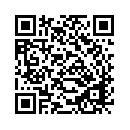Перегляд інформації про документ
Назва:
Application of fractional boundary conditions in diffraction by plane screens
Колекція:
Статті »
Автори:
Ключові слова:
Видавець:
Рік публікації:
ISBN/ISSN:
Вид документа:
стаття
Мова документа:
англійська
Додано у архів:
10.02.2016
Зведена інформація по документу:
Ivakhnychenko, M.V. Application of fractional boundary conditions in diffraction by plane screens [Текст] / Ivakhnychenko M.V., Ahmedov T.M., Veliev E.I. // Статті : - , 2010.
Постійне посилання:
Посилання на файл:
Коротке посилання на файл:
Аанотація:

вiдсутня

отсутствует

Problems of diffraction by plane screens described by fractional boundary conditions (FBC) are considered. FBC involves fractional derivative of tangential field components. FBC can be treated as intermediate case between well known boundary conditions for perfectly electric conductor (PEC) and perfectly magnetic conductor (PMC). A method to solve two-dimensional problems of scattering of E-polarized plane wave on a strip and half plane with FBC is proposed. The considered problems are reduced to coupled integral equations which are discretized using orthogonal polynomials. The method allows to obtain physical characteristics with any desired accuracy. One important feature of the considered integral equations is noted: these equations can be solved analytically for one special value of the fractional order equal to 0.5 for any frequency.© 2019 — Розроблено лабораторією інформаційно-пошукових систем НТУ "ХПІ"